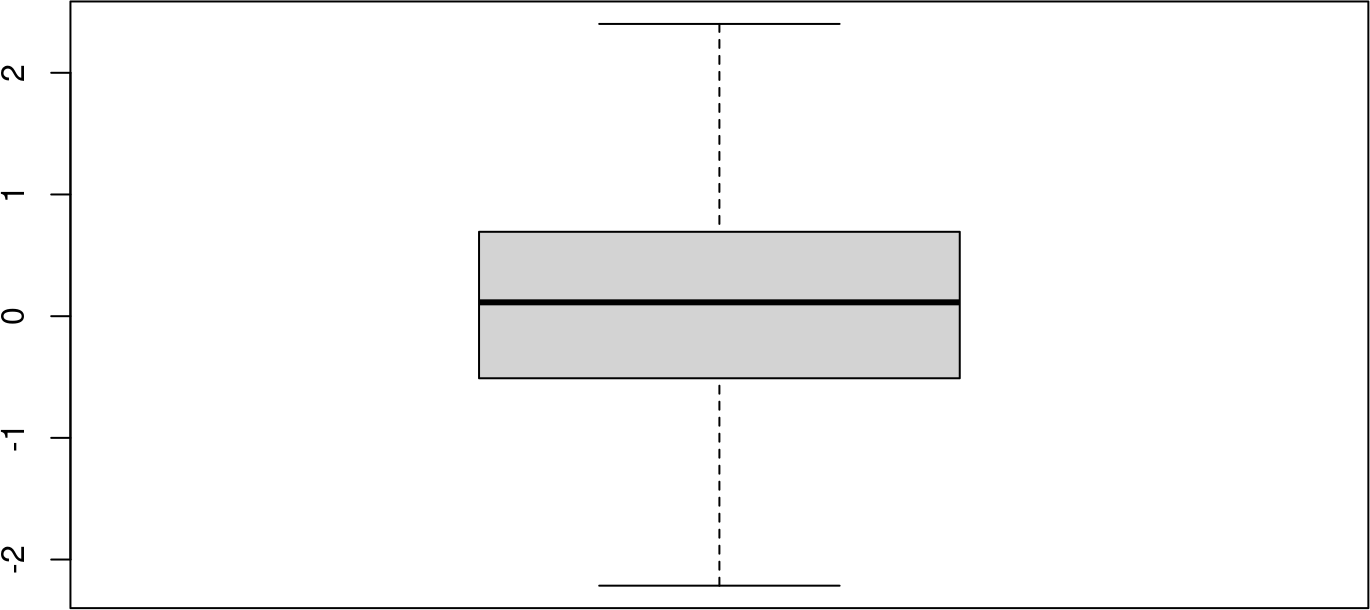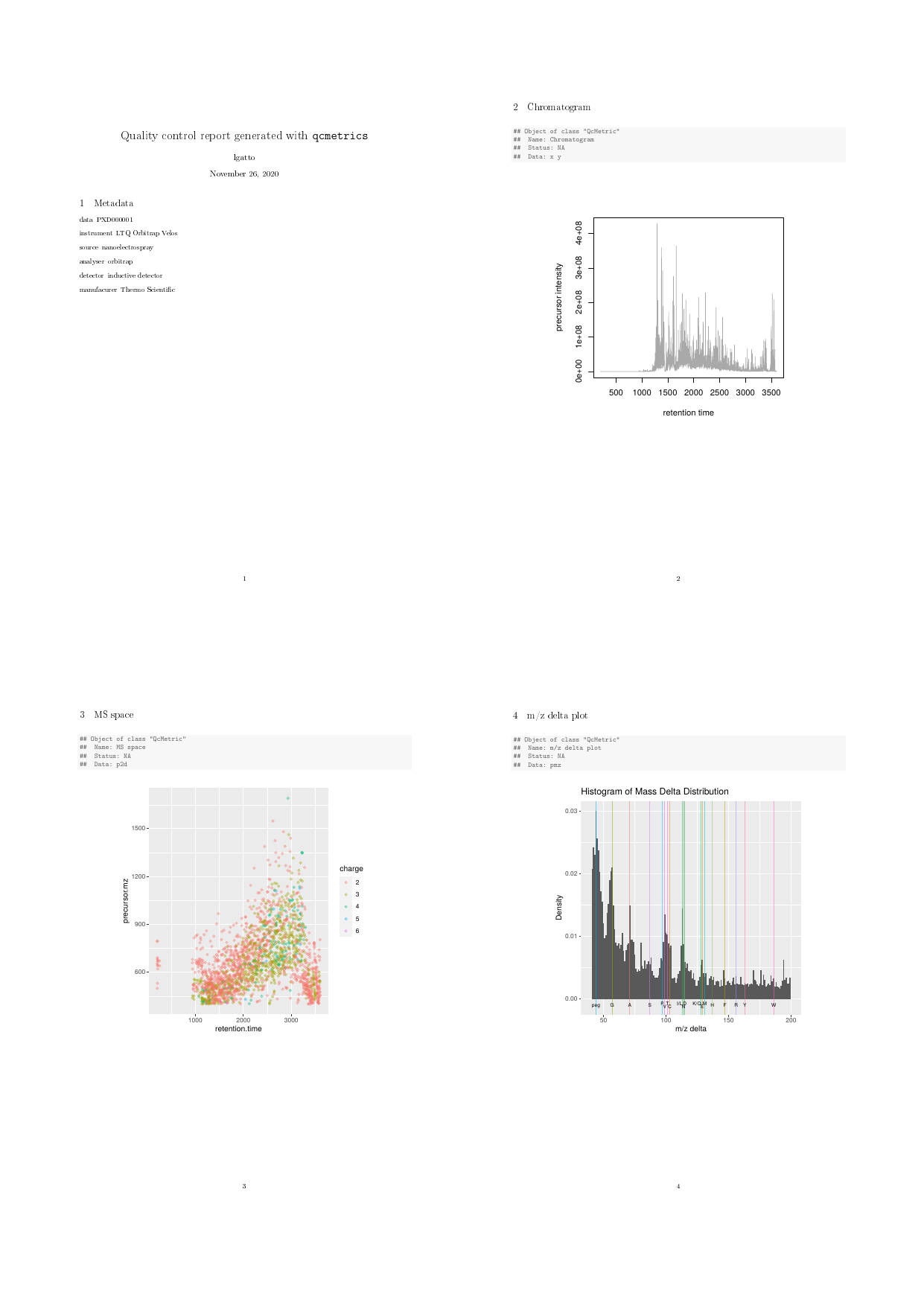# 1 Introduction

Quality control (QC) is an essential step in any analytical process. Data of poor quality can at best lead to the absence of positive results or, much worse, false positives that stem from uncaught faulty and noisy data and much wasted resources in pursuing red herrings.

Quality is often a relative concept that depends on the nature of the biological sample, the experimental settings, the analytical process and other factors. Research and development in the area of QC has generally lead to two types of work being disseminated. Firstly, the comparison of samples of variable quality and the identification of metrics that correlate with the quality of the data. These quality metrics could then, in later experiments, be used to assess their quality. Secondly, the design of domain-specific software to facilitate the collection, visualisation and interpretation of various QC metrics is also an area that has seen much development. QC is a prime example where standardisation and automation are of great benefit. While a great variety of QC metrics, software and pipelines have been described for any assay commonly used in modern biology, we present here a different tool for QC, whose main features are flexibility and versatility. The `qcmetrics` package is a general framework for QC that can accommodate any type of data. It provides a flexible framework to implement QC items that store relevant QC metrics with a specific visualisation mechanism. These individual items can be bundled into higher level QC containers that can be readily used to generate reports in various formats. As a result, it becomes easy to develop complete custom pipelines from scratch and automate the generation of reports. The pipelines can be easily updated to accommodate new QC items of better visualisation techniques.

Section 2 provides an overview of the framework. In section 3, we use proteomics data (subsection 3.2) to demonstrate the elaboration of QC pipelines: how to create individual QC objects, how to bundle them to create sets of QC metrics and how to generate reports in multiple formats. We also show how the above steps can be fully automated through simple wrapper functions. Although kept simple in the interest of time and space, these examples are meaningful and relevant. In section 4, we provide more detail about the report generation process, how reports can be customised and how new exports can be contributed. We proceed in section 4.3 to the consolidation of QC pipelines using R and elaborate on the development of dedicated QC packages with `qcmetrics`.

# 2 The QC classes

The package provides two types of QC containers. The `QcMetric` class stores data and visualisation functions for single metrics. Several such metrics can be bundled into `QcMetrics` instances, that can be used as input for automated report generation. Below, we will provide a quick overview of how to create respective `QcMetric` and `QcMetrics` instances. More details are available in the corresponding documentations.

## 2.1 The `QcMetric` class

A QC metric is composed of a description (`name` in the code chunk below), some QC data (`qcdata`) and a `status` that defines if the metric is deemed of acceptable quality (coded as `TRUE`), bad quality (coded as `FALSE`) or not yet evaluated (coded as `NA`). Individual metrics can be displayed as a short textual summary or plotted. To do the former, one can use the default `show` method.

``````library("qcmetrics")
qc <- QcMetric(name = "A test metric")
qcdata(qc, "x") <- rnorm(100)
qcdata(qc) ## all available qcdata``````
``##  "x"``
``summary(qcdata(qc, "x")) ## get x``
``````##    Min. 1st Qu.  Median    Mean 3rd Qu.    Max.
## -2.2147 -0.4942  0.1139  0.1089  0.6915  2.4016``````
``show(qc) ## or just qc``
``````## Object of class "QcMetric"
##  Name: A test metric
##  Status: NA
##  Data: x``````
``````status(qc) <- TRUE
qc``````
``````## Object of class "QcMetric"
##  Name: A test metric
##  Status: TRUE
##  Data: x``````

Plotting `QcMetric` instances requires to implement a plotting method that is relevant to the data at hand. We can use a `plot` replacement method to define our custom function. The code inside the `plot` uses `qcdata` to extract the relevant QC data from `object` that is then passed as argument to `plot` and uses the adequate visualisation to present the QC data.

``plot(qc)``
``## Warning in x@plot(x, ...): No specific plot function defined``
``````plot(qc) <- function(object, ... ) boxplot(qcdata(object, "x"), ...)
plot(qc)``````## 2.2 The `QcMetrics` class

A `QcMetrics` object is essentially just a list of individual instances. It is also possible to set a list of metadata variables to describe the source of the QC metrics. The metadata can be passed as an `QcMetadata` object (the way it is stored in the `QcMetrics` instance) or directly as a named `list`. The `QcMetadata` is itself a `list` and can be accessed and set with `metadata` or `mdata`. When accessed, it is returned and displayed as a `list`.

``````qcm <- QcMetrics(qcdata = list(qc))
qcm``````
``````## Object of class "QcMetrics"
##  containing 1 QC metrics.
``````metadata(qcm) <- list(author = "Prof. Who",
lab = "Big lab")
qcm``````
``````## Object of class "QcMetrics"
##  containing 1 QC metrics.
``mdata(qcm)``
``````## \$author
##  "Prof. Who"
##
## \$lab
##  "Big lab"``````

The metadata can be updated with the same interface. If new named items are passed, the metadata is updated by addition of the new elements. If a named item is already present, its value gets updated.

``````metadata(qcm) <- list(author = "Prof. Who",
lab = "Cabin lab",
University = "Universe-ity")
mdata(qcm)``````
``````## \$author
##  "Prof. Who"
##
## \$lab
##  "Cabin lab"
##
## \$University
##  "Universe-ity"``````

The `QcMetrics` can then be passed to the `qcReport` method to generate reports, as described in more details below.

# 3 Creating QC pipelines

The Microarray degradation section has been removed since the packages it was depending on have been deprecated.

## 3.2 Proteomics raw data

To illustrate a simple QC analysis for proteomics data, we will download data set `PXD00001` from the ProteomeXchange repository in the mzXML format (Pedrioli and others 2004). The MS2 spectra from that mass-spectrometry run are then read into Rand stored as an `MSnExp` experiment using the `readMSData` function from the `MSnbase` package (Gatto and Lilley 2012).

``````library("RforProteomics")
msfile <- getPXD000001mzXML()
library("MSnbase")
exp <- readMSData(msfile, verbose = FALSE)``````

In the interest of time, this code chunk has been pre-computed and a subset (1 in 3) of the `exp` instance is distributed with the package. The data is loaded with

``load(system.file("extdata/exp.rda", package = "qcmetrics"))``

The `QcMetrics` will consist of 3 items, namely a chromatogram constructed with the MS2 spectra precursor’s intensities, a figure illustrating the precursor charges in the MS space and an m/z delta plot illustrating the suitability of MS2 spectra for identification (see `?plotMzDelta` or (Foster et al. 2011)).

``````qc1 <- QcMetric(name = "Chromatogram")
x <- rtime(exp)
y <- precursorIntensity(exp)
o <- order(x)
qcdata(qc1, "x") <- x[o]
qcdata(qc1, "y") <- y[o]
plot(qc1) <- function(object, ...)
plot(qcdata(object, "x"),
qcdata(object, "y"),
col = "darkgrey", type ="l",
xlab = "retention time",
ylab = "precursor intensity")``````
``````qc2 <- QcMetric(name = "MS space")
qcdata(qc2, "p2d") <- plot2d(exp, z = "charge", plot = FALSE)
plot(qc2) <- function(object) {
require("ggplot2")
print(qcdata(object, "p2d"))
}``````
``````qc3 <- QcMetric(name = "m/z delta plot")
qcdata(qc3, "pmz") <- plotMzDelta(exp, plot = FALSE,
verbose = FALSE)
plot(qc3) <- function(object)
suppressWarnings(print(qcdata(object, "pmz")))``````

Note that we do not store the raw data in any of the above instances, but always pre-compute the necessary data or plots that are then stored as `qcdata`. If the raw data was to be needed in multiple `QcMetric` instances, we could re-use the same `qcdata` environment to avoid unnecessary copies using `qcdata(qc2) <- qcenv(qc1)` and implement different views through custom `plot` methods.

Let’s now combine the three items into a `QcMetrics` object, decorate it with custom metadata using the MIAPE information from the `MSnExp` object and generate a report.

``````protqcm <- QcMetrics(qcdata = list(qc1, qc2, qc3))
data = "PXD000001",
instrument = experimentData(exp)@instrumentModel,
source = experimentData(exp)@ionSource,
analyser = experimentData(exp)@analyser,
detector = experimentData(exp)@detectorType,
manufacurer = experimentData(exp)@instrumentManufacturer)``````

The status column of the summary table is empty as we have not set the QC items statuses yet.

``qcReport(protqcm, reportname = "protqc")``Figure 1: Proteomics QC report

The complete pdf report is available with:

``browseURL(example_reports("protqc"))``

## 3.3 Processed N15 labelling data

In this section, we describe a set of N15 metabolic labelling QC metrics (Krijgsveld et al. 2003). The data is a phospho-enriched N15 labelled Arabidopsis thaliana sample prepared as described in (Groen et al. 2013). The data was processed with in-house tools and is available as an `MSnSet` instance. Briefly, MS2 spectra were search with the Mascot engine and identification scores adjusted with Mascot Percolator. Heavy and light pairs were then searched in the survey scans and N15 incorporation was estimated based on the peptide sequence and the isotopic envelope of the heavy member of the pair (the `inc` feature variable). Heavy and light peptides isotopic envelope areas were finally integrated to obtain unlabelled and N15 quantitation data. The `psm` object provides such data for PSMs (peptide spectrum matches) with a posterior error probability < 0.05 that can be uniquely matched to proteins.

We first load the `MSnbase` package (required to support the `MSnSet` data structure) and example data that is distributed with the `qcmetrics` package. We will make use of the `ggplot2` plotting package.

``````library("ggplot2")
library("MSnbase")
data(n15psm)
psm``````
``````## MSnSet (storageMode: lockedEnvironment)
## assayData: 1772 features, 2 samples
##   element names: exprs
## protocolData: none
## phenoData: none
## featureData
##   featureNames: 3 5 ... 4499 (1772 total)
##   fvarLabels: Protein_Accession Protein_Description ... inc (21 total)
## experimentData: use 'experimentData(object)'
##   pubMedIds: 23681576
## Annotation:
## - - - Processing information - - -
## Subset [22540,2][1999,2] Tue Sep 17 01:34:09 2013
## Removed features with more than 0 NAs: Tue Sep 17 01:34:09 2013
## Dropped featureData's levels Tue Sep 17 01:34:09 2013
##  MSnbase version: 1.9.7``````

The first QC item examines the N15 incorporation rate, available in the `inc` feature variable. We also defined a median incorporation rate threshold `tr` equal to 97.5 that is used to set the QC status.

``````## incorporation rate QC metric
qcinc <- QcMetric(name = "15N incorporation rate")
qcdata(qcinc, "inc") <- fData(psm)\$inc
qcdata(qcinc, "tr") <- 97.5
status(qcinc) <- median(qcdata(qcinc, "inc")) > qcdata(qcinc, "tr")``````

Next, we implement a custom `show` method, that prints 5 summary values of the variable’s distribution.

``````show(qcinc) <- function(object) {
qcshow(object, qcdata = FALSE)
cat(" QC threshold:", qcdata(object, "tr"), "\n")
cat(" Incorporation rate\n")
print(summary(qcdata(object, "inc")))
invisible(NULL)
}``````

We then define the metric’s `plot` function that represent the distribution of the PSM’s incorporation rates as a boxplot, shows all the individual rates as jittered dots and represents the `tr` threshold as a dotted red line.

``````plot(qcinc) <- function(object) {
inc <- qcdata(object, "inc")
tr <- qcdata(object, "tr")
lab <- "Incorporation rate"
dd <- data.frame(inc = qcdata(qcinc, "inc"))
p <- ggplot(dd, aes(factor(""), inc)) +
geom_jitter(colour = "#4582B370", size = 3) +
geom_boxplot(fil``````